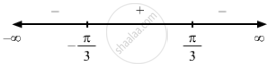PUC Karnataka Science Class 12Department of Pre-University Education, Karnataka
Share

F(X) = 2sin X − X, − π 2 < < X < π 2 . - PUC Karnataka Science Class 12 - Mathematics

Question

f(x)=2sinx-x, -pi/2<=x<=pi/2

Solution

$\text { Given: } \hspace{0.167em} f\left( x \right) = 2 \sin x - x$

$\Rightarrow f'\left( x \right) = 2 \cos x - 1$

$\text { For a local maximum or a local minimum, we must have }$

$f'\left( x \right) = 0$

$\Rightarrow 2 \cos x - 1 = 0$

$\Rightarrow \cos x = \frac{1}{2}$

$\Rightarrow x = \frac{\pi}{3} or \frac{- \pi}{3}$Sincef '(x) changes from positive to negative when x increases through $\frac{\pi}{3}$ x = $\frac{\pi}{3}$ is the point of local maxima.

The local maximum value of  f (x) at x = $\frac{\pi}{3}$ is given by $2 \sin \left( \frac{\pi}{3} \right) - \frac{\pi}{3} = \sqrt{3} - \frac{\pi}{3}$

Since f '(x) changes from negative to positive when x increases through $- \frac{\pi}{3}$ x = $- \frac{\pi}{3}$ is the point of local minima.

The local minimum value of  f (x)  at x = $- \frac{\pi}{3}$  is given by $2 \sin \left( \frac{- \pi}{3} \right) + \frac{\pi}{3} = \frac{\pi}{3} - \sqrt{3}$

Is there an error in this question or solution?

Video TutorialsVIEW ALL 

Solution F(X) = 2sin X − X, − π 2 < < X < π 2 . Concept: Graph of Maxima and Minima.
S